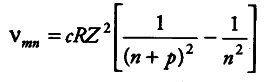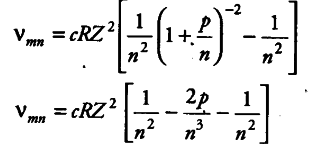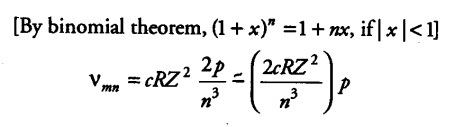# Show that the first few frequencies of light that is emitted

Show that the first few frequencies of light that is emitted when electrons fall to nth level from levels higher than n, are approximate harmonics ( i.e. in the ratio 1:2:3) when n » 1.

The frequency of any line in a series in the spectrum of hydrogen like atoms corresponding to the transition of electrons from (n + p) level to nth level can be expressed as a difference of two terms.where, m = n + p,(p = 1, 2,3, …) and R is Rydberg’s constant.
p«nThus, the first few frequencies of light that is emitted when electrons fall to the nth level from levels higher than n, are approximate harmonic (i.e. in the ratio 1:2:3 …) when n»l.